# Practical exercise 5: GAS GIANT JUPITER

Jupiter is closest to the Earth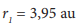and furthest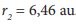. Assume that the Earth moves in a circular orbit and the orbital planes of the Earth and Jupiter merge together, see Fig. 3.

Then determine:

a) perihelion and aphelion for the orbit of Jupiter,

b) semi major and semi minor axes, distance and numerical eccentricity of the ellipse,

c) orbital period,

d) how many times Jupiter in the perihelion has a higher speed than in the aphelion.Fig. 3 for the task analysis

Solution:

a) The closest distance of Pluto from the Sun is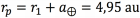.

The greatest distance is then equal to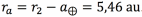.

b) For each ellipse we determine the semi major axis as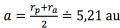, the distance eccentricity is according to the figure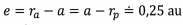, the numerical eccentricity is from the definition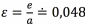, we determine the semi minor axis from the Pythagorean theorem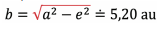.

c) We will proceed similarly as in Task IV. If we want to use the form of the 3rd Kepler's law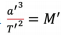, tak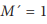, then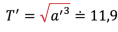. The period is therefore =11,9 rokov

d) It follows from the 2nd Kepler's law that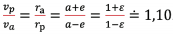.